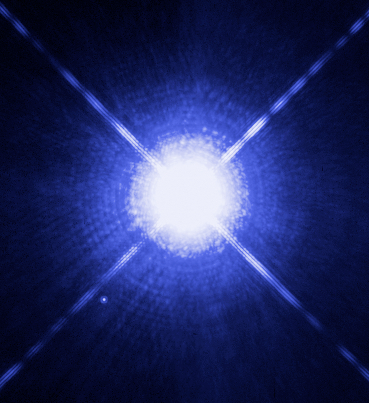# Difference Between Absolute and Apparent Magnitude

## Main Difference – Absolute vs. Apparent Magnitude

Absolute and apparent magnitudes measure the brightness of astronomical objects. The main difference between absolute and apparent magnitude is that apparent magnitude measures the observed brightness of an object from any point, whereas absolute magnitude calculates the brightness of the object as seen from a standard distance away from the object.

## What is Apparent Magnitude

Apparent magnitude is a measurement of how bright an object seems at the point where it is observed. It expresses brightness on a scale, giving brighter objects lower values and fainter objects higher values. The Sun, obviously, is the brightest object in the sky when observed from Earth, so it has the lowest apparent magnitude value of -26.7. The faintest objects that a human eye can detect have apparent magnitudes of about 6. The Hubble Telescope can detect objects having an apparent magnitude of up to 31.5.

## What is Absolute Magnitude

Apparent magnitude is not by itself useful to astronomers who are studying stars. A relatively faint star might appear brighter to observers on Earth if it is simply situated closer to the Earth, whereas a distant star, which is in fact very luminous, might seem faint. An obvious example is the Sun itself. Sirius is a star which is comparatively much more luminous than the Sun. However, because it is much farther away from Earth, it appears much fainter.Sirius A, as seen from the Hubble telescope

Therefore, it is, clearly, more useful to have a scale that can compare the actual brightness of celestial objects. This is the purpose of absolute magnitude. The absolute magnitude of an object is defined as the brightness of an object at a distance of 10 parsecs away from it. (A parsec is a unit used to measuring distances between stars. A parsec is about 3.26 light years, and the distance between the Sun and Proxima Centauri, the closest star to the Sun is about 1.3 parsecs).

If the apparent magnitude of a star at a distance$d$ parsecs away from us is given by$m$, then the absolute magnitude$M$ is calculated from apparent magnitude using the following formula:$M=m-5\mathrm{log_{10}\left( \frac{d}{10}\right)}$

From the definition of absolute magnitude, the absolute magnitude is equal to the apparent magnitude when we observe the object from 10 parsecs away. This is confirmed by the equation. When$d=10\mathrm{\:pc}$, the log term becomes$\mathrm{-5log_{10}\left( 1\right)=0}$, making$M=m$.

## Difference between Absolute and Apparent Magnitude

### Measurement

Apparent magnitude gives the brightness of an object, observed from any point.

Absolute magnitude gives the brightness of an object as seen from 10 parsecs away.

Image Courtesy

“This Hubble Space Telescope image shows Sirius A, the brightest star in our nighttime sky, along with its faint, tiny stellar companion, Sirius B…” by NASA, ESA, H. Bond (STScI), and M. Barstow (University of Leicester) [CC BY-SA 3.0], via Wikimedia Commons